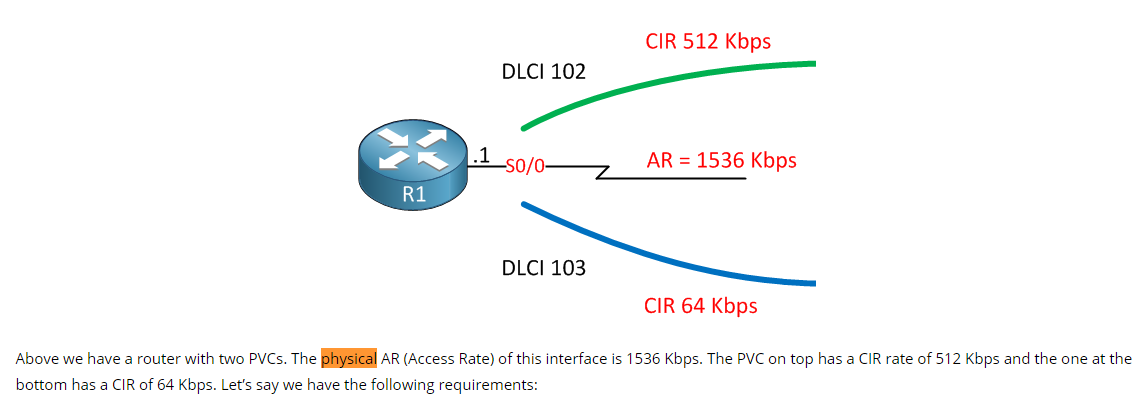# Shaping with Burst up to interface speed

This topic is to discuss the following lesson:

Thanks a lot Rene. Excellent post !!!

Thanks for this clear and concise lesson, much appreciated

3.200 50ms: How did you get the 3.200?
Thanks.

Hi Paul,

In my example, the Tc of 50 ms is a requirement.

The bottom link has a CIR of 64kbps, that’s 64000 bits per second (1000ms).

64000 bits = 1000ms

Divide it by 10 and we get:

6400 bits = 100ms

To get to 50ms, divide it by 2:

3200 bits = 50 ms.

Rene

Hi, Rene

What do you mean the physical access rate? For me it is not clear, could give an explanation on this?

Hello Stefanio.

When Rene refers to the physical access rate, he is referring to the maximum speed available on the physical interface. In this specific case:Rene is referring to the maxiumum speed that the Serial interface S0/0 is capable of providing.

I hope this has been helpful!

Laz

Hi Rene,

That was a great bunch of posts. Just for the benefit of students, can you please state this?

Bc and Be - are they supposed to be expressed in bits, bytes ? vary with different families/models of Cisco devices?

Thanks,
Ayyappan

Hello Ayyappan

Looking at the below output of the context sensitive help, we can see that both Bc and Be are measured in bits:

This image is from the following lesson:

Now Rene doesn’t mention which platform or which IOS he is using, however, it should be the same for most platforms.

I hope this has been helpful!

Laz

Hi Rene and team,
This sounds like an interesting topic. But I actually did not get any formula in all the calculations you made. All I could figure out is how you convert Kbps to bps. could you please help with a formula on how you came out with those figures? what does Be stands for?
Thank you for all you do

Hello Ayong

Here’s a summary of the mathematics:

• CIR is the committed information rate, which is the number of bits per second which is the data rate agreed upon between the ISP and the customer.
• Tc is the time interval used for calculations. In this lesson, Tc = 50ms
• Bc is the committed burst rate. This is the number of bits that will be sent every Tc, based on the CIR
• Be is the excess burst rate, which is the additional bits per Tc that we will allow under certain conditions. In this lesson, the Be is the difference between the interface rate (AR) and Bc.
• The AR is the access rate, which in this lesson is 1536 Kbps or 1,536,000 bps.

In the lesson, Tc = 50ms, and CIR = 512 Kbps = 512,000 bps for DLCI 102. Therefore:

• there are 1000 ms / 50 ms = 20 Tcs within a second
• Bc = CIR / 20 = 512,000 bps / 20 = 25,600 bits per Tc
• Be = AR - Bc = 1,536,000 bps -

Now how many bits are sent every Tc at the AR rate, that is, the rate of the interface itself? Since Tc = 50ms, we use the same logic as above, and we do the following:

• there are 1000 ms / 50 ms = 20 Tcs within a second
• Bits sent every Tc at the AR rate = 1,536,000 bps / 20 = 76800 bits per Tc
• Be = 76,800 bits per Tc - Bc = 76,800 bits per Tc - 25,600 bits per Tc = 51,200 bits

You can follow the same logic to find the Be for the other DLCI.

I hope this has been helpful!

Laz

Laz,
Thank you so much. That is exactly what I need for this topic. I appreciate it.
Best.

1 Like